# 深刻剖析与实战BCELoss详解（主）和BCEWithLogitsLoss（次）以及与普通CrossEntropyLoss的区别（次）

### 前言

import torch
import torch.nn as nn
import torch.nn.functional as tnf


#标准形式
loss_function=nn.BCELoss()
loss_function(pred,label)
#或者
tnf.binary_cross_entropy(pred,lable)


BCEWithLogitsLoss对应
tnf.binary_cross_entropy_with_logits

CrossEntropyLoss对应
tnf.cross_entropy



### BCELoss原理

BCE:binary cross entropy。所以，其也是交叉熵的一个应用，但针对的是二分类，所以有其特殊形式。

l o s s ( x , y ) = − [ y log ⁡ x + ( 1 − y ) log ⁡ ( 1 − x ) ] loss(x,y)=-[y\log x+(1-y)\log (1-x)]

### BCELoss实操

#### 二分类情况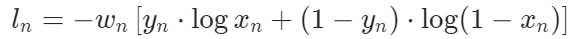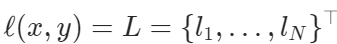data_input=torch.Tensor([0.3980, 0.8603, 0.1073])#3个样本的二分类问题，批大小是3.
data_target=torch.Tensor([0., 1., 1.])
loss=nn.BCELoss(reduction="none")#reduction="none"得到的是loss向量
loss(data_input,data_target)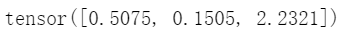print(-np.log(1-0.3980))#带原理部分的公式计算第一个样本的loss。
print(-np.log(0.8603))
print(-np.log(0.1073))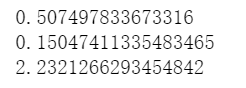loss_sum=nn.BCELoss(reduction="sum")
loss_mean=nn.BCELoss()#默认
print(loss_sum(data_input,data_target))
print(loss_mean(data_input,data_target))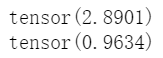loss_weight_none=nn.BCELoss(reduction="none",weight=torch.Tensor([1,1,2]))
print(loss_weight_none(data_input,data_target))#得到loss向量。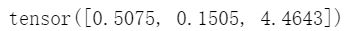import torch
import torch.nn as nn
data_input=torch.Tensor([[0.3980, 0.8603, 0.1073],[0.3980, 0.8603, 0.1073]])#一共6个样本，2批。
data_target=torch.Tensor([[0., 1., 1.],[0., 1., 1.]])
loss=nn.BCELoss(reduction="none")
loss(data_input,data_target)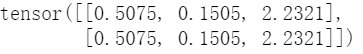#### 多分类情况

data_input=torch.Tensor([[0.3980, 0.8603, 0.1073],[0.3980, 0.8603, 0.1073]])#一共6个样本，2批。
data_target=torch.Tensor([[0., 1., 1.],[0., 1., 1.]])


1. 有2批，批大小为3，一共6个样本。
2. 模型输出，或者叫做样本输出是一个数。

1. 有1批，批大小为2，一共2个样本，一个样本的输出是一个向量，维度是3，表示是3分类问题。
2. 对于模型输出的向量x，其每一个分量值需要在0-1之间，所以我们使用sigmoid进行激活就会在这个区间。在多分类的解释下，并不需要归一化，因此我们就用sigmoid，不需要使用softmax。
3. 此时 w i w_i 解释为类别的权重，重视某个类别，则加大该类别权重即可。假设得到一个样本输出(sigmoid后的）为 x = ( x 1 , . . . , x N ) x=(x_1,...,x_N) ，要计算其loss，还是使用之前的公式：import torch
import torch.nn as nn
data_input=torch.Tensor([[0.3980, 0.8603, 0.1073],[0.3980, 0.8603, 0.1073]])#三分类问题，批大小为2.只有一个批。
data_target=torch.Tensor([[0., 1., 1.],[0., 1., 1.]])#都属于类别1，属于哪个类别，该类别就是0.
loss=nn.BCELoss(reduction="none")
loss(data_input,data_target)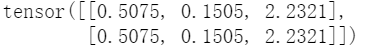### CrossEntropyLoss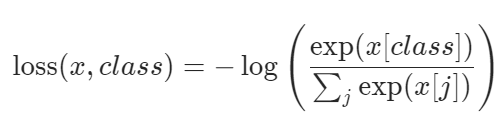loss=nn.CrossEntropyLoss()
data_input=torch.Tensor([[0.3980, 0.8603, 0.1073]])#一个批，批里面只有一个样本。
data_target=torch.Tensor().long()#这个样本属于第一个类别。
loss(data_input,data_target)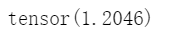-np.log(np.exp(0.3980)/(np.exp(0.3980)+np.exp(0.8603)+np.exp(0.1073)))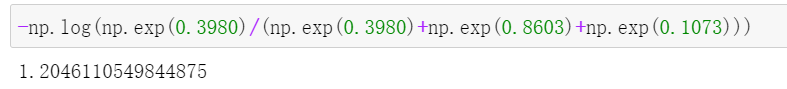### BCEWithLogitsLoss

input = torch.randn(3)#随机生成一个输入，没有被sigmoid。
target=torch.Tensor([0., 1., 1.])
loss1=nn.BCELoss()
loss2=nn.BCEWithLogitsLoss()
print("BCELoss:",loss1(torch.sigmoid(input), target))#需要sigmod
print("BCEWithLogitsLoss:",loss2(input,target))#不需要sigmoid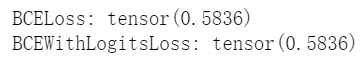06-114118
04-151070
10-081672
03-106万+
08-199466
05-175528
01-079704
03-073832

### “相关推荐”对你有帮助么？

•非常没帮助
•没帮助
•一般
•有帮助
•非常有帮助被折叠的  条评论 为什么被折叠?到【灌水乐园】发言¥2 ¥4 ¥6 ¥10 ¥20余额支付 (余额：-- )扫码支付获取中扫码支付点击重新获取扫码支付1.余额是钱包充值的虚拟货币，按照1:1的比例进行支付金额的抵扣。
2.余额无法直接购买下载，可以购买VIP、C币套餐、付费专栏及课程。余额充值